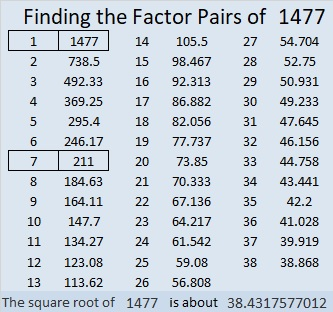# 1477 Challenge

Contents

### Today’s Puzzle:

It’s been a few days since I’ve last looked at this puzzle, but I was still able to solve it in 17 minutes. Can you beat my time?Print the puzzles or type the solution in this excel file: 12 Factors 1468-1477

### Factors of 1477:

• 1477 is a composite number.
• Prime factorization: 1477 = 7 × 211
• 1477 has no exponents greater than 1 in its prime factorization, so √1477 cannot be simplified.
• The exponents in the prime factorization are 1, and 1. Adding one to each exponent and multiplying we get (1 + 1)(1 + 1) = 2 × 2 = 4. Therefore 1477 has exactly 4 factors.
• The factors of 1477 are outlined with their factor pair partners in the graphic below.### Another Fact about the Number 1477:

1477 is the difference of two squares in two different ways:
739² – 738² = 1477
109² – 102² = 1477

This site uses Akismet to reduce spam. Learn how your comment data is processed.